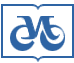## Publishing House SB RAS:

Address of the Publishing House SB RAS:
Morskoy pr. 2, 630090 Novosibirsk, Russia

# Home – Home – Jornals – Siberian Journal of Numerical Mathematics 2017 number 4

## Siberian Journal of Numerical Mathematics

### 2017 year, number 4

#### An approximation scheme for a problem of finding a subsequence

A.V. Kelmanov1,2, S.M. Romanchenko1, S.A. Khamidullin1
1Sobolev Institute of Mathematics SB RAS, 4 Acad. Koptyug avenue, Novosibirsk, Russia, 630090
2Novosibirsk State University, Pirogova st., 2, Novosibirsk, 630090, Russia
Keywords: последовательность, евклидово пространство, минимум суммы квадратов расстояний, -трудность, FPTAS, euclidean space, sequence, minimum sum of squared distances, -hardness, FPTAS

Abstract

We consider a strongly -hard Euclidean problem of finding a subsequence in a finite sequence. The criterion of the solution is a minimum sum of squared distances from the elements of a sought subsequence to its geometric center (centroid). It is assumed that a sought subsequence contains a given number of elements. In addition, a sought subsequence should satisfy the following condition: the difference between the indices of each previous and subsequent points is bounded with given lower and upper constants. We present an approximation algorithm of solving the problem and prove that it is a fully polynomial-time approximation scheme (FPTAS) when the space dimension is bounded by a constant.